# US4189212A - Wide angle zoom lens system - Google Patents

## Info

Publication number
US4189212A
US4189212A US05/793,593 US79359377A US4189212A US 4189212 A US4189212 A US 4189212A US 79359377 A US79359377 A US 79359377A US 4189212 A US4189212 A US 4189212A
Authority
US
United States
Prior art keywords
sub
lens
represent
lenses
lens component
Prior art date
Legal status (The legal status is an assumption and is not a legal conclusion. Google has not performed a legal analysis and makes no representation as to the accuracy of the status listed.)
Application number
US05/793,593
Inventor
Norio Mizutani
Soichi Nakamura
Current Assignee (The listed assignees may be inaccurate. Google has not performed a legal analysis and makes no representation or warranty as to the accuracy of the list.)
Nikon Corp
Original Assignee
Nippon Kogaku KK
Priority date (The priority date is an assumption and is not a legal conclusion. Google has not performed a legal analysis and makes no representation as to the accuracy of the date listed.)
Filing date
Publication date
Priority to JP51/50792 priority Critical
Priority to JP5079276A priority patent/JPS594681B2/ja
Priority to JP51/127766 priority
Priority to JP12776676A priority patent/JPS594684B2/ja
Application filed by Nippon Kogaku KK filed Critical Nippon Kogaku KK
Application granted granted Critical
Publication of US4189212A publication Critical patent/US4189212A/en
Assigned to NIKON CORPORATION, 2-3, MARUNOUCHI 3-CHOME, CHIYODA-KU, TOKYO, JAPAN reassignment NIKON CORPORATION, 2-3, MARUNOUCHI 3-CHOME, CHIYODA-KU, TOKYO, JAPAN CHANGE OF NAME (SEE DOCUMENT FOR DETAILS). EFFECTIVE APR. 1, 1988 Assignors: NIPPON KOGAKU, K.K.
Anticipated expiration legal-status Critical
Expired - Lifetime legal-status Critical Current

• 230000005499 meniscus Effects 0.000 claims abstract description 30
• 230000000694 effects Effects 0.000 claims abstract description 10
• 230000003287 optical Effects 0.000 claims abstract description 7
• 230000004075 alteration Effects 0.000 description 39
• 238000010276 construction Methods 0.000 description 5
• 239000011521 glass Substances 0.000 description 5
• 238000005452 bending Methods 0.000 description 3
• 210000001747 Pupil Anatomy 0.000 description 2
• 238000009877 rendering Methods 0.000 description 2
• 101700041492 CYS3 Proteins 0.000 description 1
• 206010010071 Coma Diseases 0.000 description 1
• 230000002411 adverse Effects 0.000 description 1
• 230000003247 decreasing Effects 0.000 description 1
• 230000004048 modification Effects 0.000 description 1
• 238000006011 modification reaction Methods 0.000 description 1
• 101700042500 str6 Proteins 0.000 description 1

## Images

•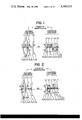•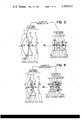•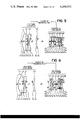•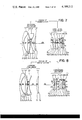•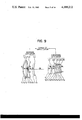•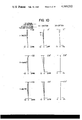•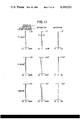•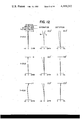•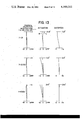•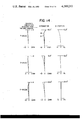•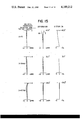•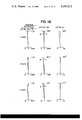••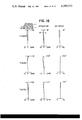## Classifications

• GPHYSICS
• G02OPTICS
• G02BOPTICAL ELEMENTS, SYSTEMS OR APPARATUS
• G02B15/00Optical objectives with means for varying the magnification
• G02B15/14Optical objectives with means for varying the magnification by axial movement of one or more lenses or groups of lenses relative to the image plane for continuously varying the equivalent focal length of the objective
• G02B15/142Optical objectives with means for varying the magnification by axial movement of one or more lenses or groups of lenses relative to the image plane for continuously varying the equivalent focal length of the objective having two groups only
• G02B15/1425Optical objectives with means for varying the magnification by axial movement of one or more lenses or groups of lenses relative to the image plane for continuously varying the equivalent focal length of the objective having two groups only the first group being negative

## Abstract

In a wide angle zoom lens system comprising a diverging lens group and a converging lens group which are both movable on the optical axis of the lens system to vary the air space between the two groups so that the magnification of the image is variable while the image plane is being maintained at a fixed position, the diverging lens group comprises, in order from the object side, a negative meniscus lens component convex to the object side, a positive lens component, a negative lens component, a positive meniscus lens component convex to the object side, the four lens components being separate from one another, and an air chamber having a converging effect being formed between the second and the third lens component, the third and the fourth lens component being so disposed that the air space therebetween is greater than the center thickness of the third member, and the converging lens group comprises at least four positive lens components a positive lens component out of which is closest to the object side and a positive lens component out of which is closest to the image side, and a negative lens component disposed among the at least four positive lens components, these five lens components being separate from one another.

## Description

BACKGROUND OF THE INVENTION
1. Field of the Invention
This invention relates to a zoom lens system, and more particularly to a wide angle zoom lens system.
2. Description of the Prior Art
As a zoom lens system covering a wide angle of view, there is known a zoom lens system which comprises two forward and rearward lens groups, namely, a diverging lens group and a converging lens group, but such zoom lens system suffers from various difficulties still left to be generally overcome, such as the destruction of the balance of spherical aberration and coma attributable to the variation in focal length, the pronounced negative distortion at the short focal length side, the variation in distortion during the focal length condition at the opposite ends of zooming, the imbalance of the chromatic aberration of magnification attributable to the angle of view, the mismatching of the curvature of image plane attributable to chromatic difference, the difficulty encountered in reducing the size of the forward lens diameter to make the entire system more compact.
These difficulties come from the fact that the forward group is a diverging lens system, and some attempts to overcome these disadvantages have heretofore been made by the use of various diverging lens systems.
For example, as far as the distortion is concerned, success has been attained in reducing the distortion by using a positive meniscus lens, convex to the object side, as a first component forming the diverging lens system, but since the first component is a positive lens, oblique rays are intensely refracted by the first component and the rays emergent therefrom form greater angles with the optical axis and therefore, if the diaphragm position is regarded as being fixed, a greater angle of view requires the oblique rays entering the first component to pass through positions more distant from the optical axis and this has prevented the forward lens diameter from being sufficiently decreased.
There is also a zoom lens system in which the first component closest to the object side is comprised of a negative meniscus lens to thereby reduce the angles of deviation of oblique rays and correct the distortion, and this is considerably effective to achieve the purpose of reducing the forward lens diameter, but the residual distortion in such system exceeds -4% at the short focal length side having a half angle of view of the order of 31°, and this could not be sufficiently practical and has been considerably inferior to the fixed focus lens having a single focal length.
SUMMARY OF THE INVENTION
It is an object of the present invention to eliminate the above-noted disadvantages and to provide a zoom lens system in which various aberrations are corrected in a well-balanced manner while distortion is maintained at a level equivalent to that in a single focal length lens and in which the forward lens diameter is reduced to thereby make the entire system compact. It is a further object of the present invention to provide such a zoom lens system covering a wide angle of view and in which even a high degree of chromatic aberration is sufficiently corrected.
The lens system according to the present invention comprises two lens groups, namely, a diverging lens group forming the forward group and a converging lens group forming the rearward group, as viewed from the object side. The two lens groups are movable on the optical axis to vary the air space between the two groups and thereby change the magnification. The basic lens shape is such that the diverging lens group which is the forward group comprises, in order from the object side, a first member which is a negative meniscus lens component convex to the object side, a second member which is a positive lens component, a third member which is a negative lens component, and a fourth member which is a positive meniscus lens component convex to the object side, these four lens components being separate from one another, and an air chamber having a converging effect is formed between the second and the third member, and the third and fourth members are so disposed that the air chamber therebetween has a greater thickness than the center thickness of the third member. The converging lens group which is the rearward group comprises, in order from the object side, a fifth member which is a positive lens component, a sixth component which is a positive lens, a seventh member which is a positive meniscus lens component convex to the object side, an eighth member which is a negative lens component, and a ninth member which is a positive lens component, these five components being separate from one another.
The invention will become more fully apparent from the following detailed description thereof taken in conjunction with the accompanying drawings.
BRIEF DESCRIPTION OF THE DRAWINGS
FIGS. 1 to 9 are cross-sectional views showing the lens arrangements according to Examples 1 to 9, respectively, of the present invention.
FIGS. 10 to 18 are graphs illustrating various aberrations in Examples 1 to 9, respectively.
DESCRIPTION OF THE PREFERRED EMBODIMENTS
Significances of the arrangement of these lens components will hereinafter be described. In the type wherein a negative meniscus lens is disposed at that side of the diverging lens group which is closest to the object, correction of the negative distortion is difficult to do, as already noted, but according to the present invention, such negative distortion is overcome by the third member or the negative lens component L3 satisfying the following condition to thereby form an air lens having a converging effect between the negative lens component L3 and the second member or the positive lens component L2 :
`0.5<(R.sub.5 +R.sub.6)/(R.sub.5 -R.sub.6)<1.2              (1),`
where R5 represents the curvature radius of the surface of the negative lens component of the third member which faces the object side, and R6 the curvature radius of the surface of the same lens component which faces the image side. It has been found that if the lower limit is exceeded, the curvature radius of the concave surface with respect to the object side will be sharper so that the achromatizing effect between this negative lens component L3 and the positive lens component L2 disposed ahead thereof will be greater, while the negative distortion at the short focal length side of the lens system will be sharply aggravated. If the upper limit is exceeded, the curvature radius of the surface facing to the image side of the negative lens component L3 will be too sharp and this will offer inconvenience to the correction of the spherical aberration at the long focal length side of the lens system. Therefore, this negative lens component L3 must satisfy the foregoing condition. Also, the negative meniscus lens component of the first member L1 and the negative lens component of the third member L3 must satisfy the relation that 0.7<|R2 |/|R6 |<1.6, where R2 and R6 represent the curvature radii of the successive surfaces, as viewed from the object side, of these two diverging lens components which face the image side, in order to perform the auxiliary role for reducing the distortion and to balance the other aberrations with one another. Especially, if this condition is departed from, it will become difficult to well correct the curvature of the image plane at the short focal length side of the lens system.
Further, if the air space D between the negative lens component of the third member L3 and the positive meniscus lens of the fourth member L4 is made greater than the center thickness of the third member L3 and if the total focal length of the diverging lens group which is the forward group is f1, the following condition must be satisfied to thicken such diverging lens group and correct the variations both in spherical aberration and curvature of image field resulting from the variation in the focal length:
`0.1≦D/|f.sub.1 |≦0.4       (2)`
If the lower limit of this condition is exceeded, it will be impossible to correct the variations both in spherical aberration and curvature of image field resulting from the variation in focal length and, if the upper limit of the condition is exceeded, the thickness of the diverging lens group will be too great so that the back principal plane of the diverging lens group will shift toward the object side, thus rendering it impossible to secure a zoom ratio. Also, if the focal length of the positive meniscus lens L4 which is the fourth member of the diverging lens group is f14, it has been found that a condition that 1.5|f1 |<f14 <3.5|f1 | is useful to make the above condition (2) more effective and serves to play the auxiliary role for correcting the variations both in spherical aberration and curvature of image field. If the upper limit of this condition is exceeded, under-correction of the spherical aberration at the long focal length side will occur to bring about negative curvature of image field at the short focal length side. If the lower limit is exceeded, over-correction of the spherical aberration at the long focal length side will occur to bring about positive curvature of image field at the short focal length side.
In the basic construction of the diverging group as described above, it becomes possible to more sufficiently correct even the high degrees of chromatic aberration such as the imbalance of the chromatic aberration of magnification attributable to the angle of view and the mismatching of the curvature of image field attributable to the chromatic difference by selecting greater Abbe numbers of glass for the divergent components in the diverging group, namely, the first and the third member L1, L3, than the Abbe numbers of glass for the convergent components in the diverging group, namely, the second and the fourth member L2, L4 and the result is that a zoom lens having a wider angle of view can be provided. A more minute study has shown that if the Abbe numbers of glass for the negative meniscus lens component of the first member L1 and the negative lens component of the third member L3 are represented by ν and the Abbe numbers of glass for the positive lens component of the second member L2 and the positive meniscus lens component of the fourth member L4 are ν2 and ν4, respectively, satisfaction of the following two conditions is an effective means for correcting the high degrees of chromatic aberration. ##EQU1##
It is already known that the converging lens group which is the rearward group is required to be of a great relative aperture due to the forward group being a diverging lens group, but when the zoom lens of this type is to be designed as compactly as possible, it is desirable that the forward principal plane be projected forwardly of the lens as in the telephoto type. When further compactness is desired, it is necessary to intensify the power both in the diverging lens group and the converging lens group and it is also necessary to correct the shift of the Petzval sum toward the negative direction resulting therefrom.
For the reasons set forth above, the present invention employs a converging lens group which is approximate to the Sonnar type comprising the five components as already described. This converging group will hereinafter be referred to as Type I. By this, the present invention has been successful in making compact a wide angle zoom lens having a construction characteristic to the Sonnar type in which the principal point of the lens system lies forwardly of the system and which permits a great aperture, and overcoming the tendency of the Petzval sum becoming negative. In the converging lens group formed by such five components, it is desirable that the refractive indices of the sixth member or the positive lens component L6 and the seventh member or the positive meniscus lens component L7 be less than 1.65 and the refractive index of the eighth member or the negative lens component L8 be higher by 0.17 or more than said refractive indices. By adding such two conditions it is possible to bring the Petzval sum more toward the positive direction and realize further compactness without adversely affecting the other aberrations.
On the other hand, where a wider angle of view is desired, the power of the diverging lens group may be relatively intensified with respect to that of the converging lens group but in that case, the backward converging lens group must be made capable of withstanding a greater angle of view in terms of aberration. Therefore, the positive lens component which is the ninth member L9 in the converging lens group which is closest to the image side may be divided into two positive lenses L9a, L9b, whereby there may be provided the effect of rendering the distortion to the positive and the freedom of correction of the curvature of image field and thus, it is possible to provide a zoom lens which can use at a wider angle of view, say, 81.6°. This converging group will hereinafter referred to as Type II. Also, if further compactness is desired, it becomes necessary that the negative curvature of image plane created by the positive lenses located forwardly of the negative lens component of the eighth member L8 is corrected to the positive by bending of the air lens formed by the surface of this negative lens component L8 which is concave to the image side and the object-looking surface of the divided positive lens L9a closer to the object side. In order that the increase in over-correction of the spherical aberration created at the long focal distance side by such bending may be eliminated by the bending of the divided positive lens closer to the object side L9a, it is desirable that such divided positive lens closer to the object side L9a may be made into a convex meniscus form with respect to the image side.
Further, in such a construction wherein the positive lens component of the converging lens group which is closest to the image side L9 is divided into the two positive lenses L9a, L9b, the two components, namely, the positive lens component of the sixth member L6 and the positive meniscus lens component of the seventh member L7 which is convex to the object side, may be united together into a single positive meniscus lens L67 which is convex to the object side, whereby it becomes possible to provide a zoom optical system covering a half angle of view of the order of 31°. With such a construction, it becomes possible to forwardly shift the entrance pupil of the converging lens group and thus, forwardly shift the entrance pupil of the entire lens system, so that a more compact zoom optical system may be provided. This converging group will hereinafter be referred to as Type III.
In the wide angle lens system of the present invention constructed in the manner as described above, a single type of the diverging lens group is usable in common with three alternative types of the converging lens group. These may be classified as follows, for various examples which will further be described.
Type I . . . Examples 1 to 3
Type II . . . Examples 4 to 8
Type III . . . Example 9
In the present invention, the above-mentioned common diverging group forms the greatest feature and it is desirable that the focal lengths of the individual lens components of the diverging group satisfy the following conditions:
`1.2<|f.sub.11 |/|f.sub.1 |<3.8,`
`1.8<f.sub.12 /|f.sub.1 |<4.0,`
`0.4<|f.sub.13 |/|f.sub.1 <1.2 and`
`1.2<f.sub.14 /|f.sub.1 |<3.8`
where f1 represents the total focal length of the diverging lens group, f1i the focal lengths of the individual lens components in the diverging group, and the suffix i the order of the lens components from the object side. For such a power arrangement of the diverging group, it is more desirable that the shape factor of the three lens components in the diverging group, except the negative lens component of the third member, be determined as follows, whereby correction of various aberrations may become easier to do.
`1.3<Q.sub.11 <8.2,`
`0.5<Q.sub.12 <1.5 and`
`-5.1<Q.sub.14 <-1.5,`
where Q1i represents the shape factor of each lens component in the diverging group, the suffix i represents the order of each lens component from the object side, and Q is defined as
`Q=(r.sub.F +r.sub.R)/(r.sub.F -r.sub.R),`
where rF and rR represent the curvature radii of the formost and rearmost surfaces, respectively, of each lens component.
Under such a construction of the common diverging group, it is desirable that the respective types of the converging group satisfy the conditions shown below. First, for the power arrangement of each lens component, the following conditions are set:
```______________________________________
Type I      Type II       Type III
______________________________________
##STR1##
##STR2##
##STR3##
##STR4##
##STR5##
##STR6##
##STR7##
##STR8##
##STR9##
##STR10##
##STR11##
##STR12##
##STR13##
##STR14##
##STR15##
##STR16##
______________________________________
```
In the foregoing, f2 represents the total focal length of the converging group, f2i the focal length of each lens component in the converging group, and the suffix i the order of each lens component from the object side.
In each type of such power arrangement, it is further desirable to determine the shape factor of each lens component and thus, the various aberrations can be corrected easily.
```______________________________________
Type I      Type II        Type III
______________________________________
-0.9 < Q.sub.24 < -0.1
-1.3 < Q.sub.21 < -0.5
-0.9 < Q.sub.21 < -0.4
-1.0 < Q.sub.22 < -0.7
-1.5 < Q.sub.22 < -0.9
-3.4 < Q.sub.23 < -1.5
-6.2 < Q.sub.23 < -1.3
-2.4 < Q.sub.22 < -1.9
0.8 < Q.sub.24 < 1.4
0.4 < Q.sub.24 < 1.2
0.5 < Q.sub.23 < 1.0
0.4 < Q.sub.25 < 1.5
1.0 < Q.sub.24 < 1.5
0.8 < Q.sub.24 < 1.4
-1.0 < Q.sub.26 < 1.3
-4.4 < Q.sub.25 < -3.9
______________________________________
```
In the foregoing, Q2i represents the shape factor of each lens component in the converging group, and the suffix i the order of each lens component from the object side.
Thus, it has become possible to provide a wide angle zoom lens system in which the various aberrations are corrected in a well-balanced manner while the distortion is maintained equivalent to that of a single focal length lens and the diameter of the forward lens is so small that the entire lens system is compact. As to the various performances of this wide angle lens system, the angle of view during the shortest focal length condition thereof ranges from 62° to maximum 81.6°, the zoom ratio is about 2.0 and the F-number is 3.5 to 4.0, and even the high degrees of chromatic aberration are sufficiently corrected.
Various examples of the present invention will now be described in detail. In any of nine examples of the wide angle zoom lens system according to the present invention, the zoom ratio is about 2.0 and F-number is of the order to 3.5 to 4.0 Examples 1, 2 and 9 respectively cover an angle of view of 62° at the shortest focal length side, Example 7 covers an angle of view of 74° and the other examples are ultra-wide angle zoom lenses which cover 80° or more. In any of these examples, the various aberrations are well corrected and especially, the distortion is so well corrected as it is comparable to or superior to the single focal length lens and the lens shape is compact.
In the two-group zoom lens system of the present invention, the air space between the two groups and accordingly, the entire shape, become maximum during the shortest focal length condition. It may thus be said that the size of such two-group zoom lens system is smaller as the distance from the foremost lens surface to the back focal plane during the shortest focal length condition is smaller. The size of the lens system as a zoom lens must be compared with the focal length, the zoom ratio, F-number, etc. taken into account, and for reference, the ratio of the distance L from the foremost lens surface to the back focal plane during the shortest focal length condition to the shortest focal length fw will be shown with respect to each example.
```__________________________________________________________________________
Examples
1      2   3   4   5   6   7   8   9
__________________________________________________________________________
L  126.44
130.87
117.33
133.76
146.50
134.34
147.68
140.47
148.82
fw 36.02
36.02
25.00
25.04
25.00
36.02
25.50
28.65
25.50
L/fw
3.51
3.63
4.69
5.34
5.86
3.73
5.79
4.90
5.84
__________________________________________________________________________
```
Example 1 is a basic form of the zoom lens system according to the present invention. In this basic form, any desired positive lens component in the converging lens group may be formed as a doublet to thereby facilitate the correction of the axial chromatic aberration and reduce the chromatic variation in spherical aberration at the longest focal length side, and herein such effects are attained in Example 2 wherein the fifth member of the positive lens component L5 is a doublet. Numerical data in Examples 1 and 2 will be shown below, and the lens arrangement of these Examples in their shortest focal length condition are shown in FIGS. 1 and 2, while various aberrations in these Examples are illustrated in FIGS. 10 and 11.
EXAMPLE 1
Total focal length: 36.023-49.497-68.795
F-number: 3.5
Maximum angle of view: 62°
Back focal distance: 43.776-53.991-68.622
```______________________________________
r.sub.1 =50.379
d.sub.1 =1.3
n.sub.1 =1.74443
ν.sub.1 =49.4
r.sub.2 =27.469
d.sub.2 =6.3
f.sub.1 =
r.sub.3 =670.373
d.sub.3 =3.5
n.sub.2 =1.5168
ν.sub.2 =64.2
r.sub.4 =-114.009
d.sub.4 =0.1
-53.0   r.sub.5 =∞
d.sub.5 =1.8
n.sub.3 =1.713
ν.sub.3 =53.9
r.sub.6 =32.531
d.sub.6 =8.4
r.sub.7 =35.195
d.sub.7 =3.4
n.sub.4 =1.76182
ν.sub.4 =26.5
r.sub.8 =57.719
d.sub.8 =29.009
-12.916-
0.846
r.sub.9 =101.102
d.sub.9 =3.0
n.sub.5 =1.5168
ν.sub.5 =64.2
r.sub.10 =-763.611
d.sub.10 =0.1
r.sub.11 =28.387
d.sub.11 =66.4
n.sub.6 =1.52
ν.sub.6 =70.1
f.sub.2 =
r.sub.12 =-449.780
d.sub.12 =0.1
r.sub.13 =19.335
d.sub.13 =5.9
n.sub.7 =1.52
ν.sub.7 =70.1
40.182
r.sub.14 =37.463
d.sub.14 =4.0
r.sub.15 =-564.061
d.sub.15 =1.4
n.sub.8 =1.80518
ν.sub.8 =25.5
r.sub.16 =16.279
d.sub.16 =3.85
r.sub.17 =44.719
d.sub.17 =4.1
n.sub.9 =1.6727
ν.sub.9 =32.2
r.sub.18 =-56.902
______________________________________
```
In order from the object side, r1, r2, r3, . . . represent the curvature radii of the refracting surfaces of the lenses, d1, d2, d3, . . . represent the center thickness of the lenses and the air spaces therebetween, and n1, n2, n3, . . . and ν1, ν2, ν3, . . . represent the refractive indices and Abbe numbers of the lenses.
EXAMPLE 2
Total focal length: 36.023-49.497-68.796
F-number: 3.5
Maximum angle of view: 62°
Back focal distance: 43.491-52.793-66.114
```______________________________________
r.sub.1 =52.287
d.sub.1 =1.5
n.sub.1 =1.72342
.sub.1 =38.0
r.sub.2 =35.517
d.sub.2 =4.9
f.sub.1 =
r.sub.3 =∞
d.sub.3 =4.0
n.sub.2 =1.5168
.sub.2 =64.2
r.sub.4 =-98.799
d.sub.4 =0.1
-59.942 r.sub.5 =879.752
d.sub.5 =2.0
n.sub.3 =1.72
.sub.3 =50.3
r.sub.6 =27.242
d.sub.6 =8.5
r.sub.7 =34.469
d.sub.7 =3.9
n.sub.4 =1.76182
.sub.4 =26.5
r.sub.8 =61.405
d.sub.8 =33.625
-14.882-
0.825
r.sub.9 =117.209
d.sub.9 =3.5
n.sub.5 =1.62299
.sub.5 =58.1
r.sub.10 =-98.386
d.sub.10 =1.0
n.sub.6 =1.80518
.sub.6 25.5
r.sub.11 =323.279
d.sub.11 =0.1
r.sub.12 =-28.810
d.sub.12 =5.75
n=1.51118
.sub.7 =50.9
f.sub.2 =
r.sub.13 =516.415
d.sub.13 =0.1
41.379  r.sub.14 =19.119
d.sub.14 =5.2
n.sub.8 =1.51118
.sub.8 =50.9
r.sub.15 =39.311
d.sub.15 =2.9
r.sub.16 =∞
d.sub.16 =2.5
n.sub.9 =1.80518
.sub.9 =25.5
r.sub.17 =16.622
d.sub.17 =4.0
r.sub.18 =50.614
d.sub.18 =3.8
n.sub.10 =1.61293
.sub.10 =36.9
r.sub.19 =-48.431
______________________________________
```
In order from the object side, r1, r2, r3, . . . represent the curvature radii of the refracting surfaces of the lenses, d1, d2, d3, . . . represent the center thickness of the lenses and the air spaces therebetween, and n1, n2, n3, . . . and ν1, ν2, ν3, . . . represent the refractive indices and Abbe numbers of the lenes.
Example 3 is substantially identical in lens arrangement to the Example 1, but as already noted, in the diverging group thereof, the Abbe numbers of glass for the diverging lens components are selected to greater values than those for the converging lens components to thereby sufficiently corrent even the high degrees of chromatic aberration such as the imbalance of the chromatic difference of magnification attributable to the angle of view and the mismatching of the image field curvature resulting from the chromatic difference, and the result is the provision of a zoom lens covering a wider angle of view. The numerical data of this Example will be shown below, and the lens arrangement thereof during the shortest focal length condition is shown in FIG. 3 while the various aberrations therein are illustrated in FIG. 12.
EXAMPLE 3
Total focal length: 25.50-35.150-48.805
F-number: 4
Maximum angle of view: 80.6°
Back focal distance: 40.102-48.838-61.200
```______________________________________
r.sub.1 =80.0
d.sub.1 =3.5
n.sub.1 =1.77279
ν.sub.1 =49.4
r.sub.2 =32.471
d.sub.2 =10.0
f.sub.1 =
r.sub.3 =-1000.0
d.sub.3 =5.0
n.sub.2 =1.60342
ν.sub.2 =38.0
r.sub.4 =-68.877
d.sub.4 =0.1
-45.379 r.sub.5 =-429.670
d.sub.5 =3.3
n.sub.3 =1.80279
ν.sub.3 =46.8
r.sub.6 =30.696
d.sub.6 =12.9
r.sub.7 =42.617
d.sub.7 =3.6
n.sub.4 =1.71736
ν.sub.4 =29.5
r.sub.8 =93.788
d.sub.8 =38.834
-18.764-
3.925
r.sub.9 =109.554
d.sub.9 =4.2
n.sub.5 =1.53172
ν.sub.5 =48.9
r.sub.10 =-184.418
d.sub.10 =0.1
r.sub.11 =37.007
d.sub.11 =5.1
n.sub.6 =1.50137
ν.sub.6 =56.5
f.sub.2 =
r.sub.12 =-459.818
d.sub.12 =0.1
r.sub.13 =21.134
d.sub.13 =7.6
n.sub.7 =1.50137
ν.sub.7 =56.5
41.082
r.sub.14 =73.546
d.sub.14 =3.5
r.sub.15 =-154.35
d.sub.15 =2.4
n.sub.8 =1.79504
ν.sub.8 =28.4
r.sub.16 =17.663
d.sub.16 =3.54
r.sub.17 =53.968
d.sub.17 =3.8
n.sub.9 =1.46450
ν.sub.9 =65.8
r.sub.18 =-27.275
______________________________________
```
In order from the object side, r1, r2, r3, . . . represent the curvature radii of the refracting surfaces of the lenses, d1, d2, d3, . . . represent the center thickness of the lenses and the air spaces therebetween, and n1, n2, n3, . . . and ν1, ν2, ν3, . . . represent the refractive indices and Abbe number of the lenses.
Example 4 is a basic form of the present invention in which the positive lens component L9 which is the ninth member located closest to the image side in Example 1 is divided into two positive lenses L9a, L9b to thereby increase the angle of view. In this Example, by forming as a doublet the negative meniscus lens component L1 which is the first member, the negative lens component L3 which is the third member, or the positive meniscus lens component L4 which is the fourth member in the diverging lens group, it is possible to adopt a great Abbe number for the positive lens component L2 which is the second member and facilitate the correction of the high degrees of chromatic aberration. Further, by forming as a doublet the positive lens component L2 which is the second member, it will also be possible to further increase the equivalent Abbe number and facilitate the correction of the high degrees of chromatic aberration, but in the present Example, the negative lens component L3 which is the third member is formed as a doublet to provide the effect of correcting such chromatic aberration.
Examples 5 and 6 are the forms in which one of the two divided positive lens components L9a in Example 4 which is closer to the object side is formed as a doublet and this positive lens is designed with a low refractive index while the negative lens is designed with a high refractive index, thereby well correcting the Petzval sum. Again in these Examples, the effect similar to that of Example 2 is further added. The numerical data of Examples 4, 5 and 6 will be shown below, and the lens arrangements of these Examples during their shortest focal length condition are shown in FIGS. 4, 5 and 6, while the various aberrations in these Examples are illustrated in FIGS. 13, 14 and 15.
EXAMPLE 4
Total focal length: 25-35.355-47.625
F-number: 4
Maximum angle of view: 81.6°
Back focal distance: 37.831-46.655-57.134
```______________________________________
r.sub.1 =160.
d.sub.1 =3.2
n.sub.1 =1.713
ν.sub.1 =53.9
r.sub.2 =33.8
d.sub.2 =6.5
r.sub.3 =365.63
d.sub.3 =4.7
n.sub.2 =1.5168
ν.sub.2 =64.2
f.sub.1 =
r.sub.4 =-62.5
d.sub.4 =0.1
r.sub.5 =430.791
d.sub.5 =2.0
n.sub.3 =1.74
ν.sub.3 =28.2
-40.0
r.sub.6 =500.
d.sub.6 =0.7
n.sub.4 =1.713
ν.sub.4 =53.9
r.sub.7 =23.05
d.sub.7 =10.5
r.sub.8 =30.503
d.sub.8 =2.8
n.sub.5 =1.80518
ν.sub.5 =25.5
r.sub.9 =46.128 9=26.364- 10.390- 0.439
r.sub.10 =49.
d.sub.10 =1.9
n.sub.6 =1.52
ν.sub.6 =70.1
r.sub.11 =573.031
d.sub.11 =0.1
r.sub.12 =26.375
d.sub.12 =6.3
n.sub.7 =1.52
ν.sub.7 =70.1
r.sub.13 =220.
d.sub.13 =0.1
r.sub.14 =18.845
d.sub.14 =3.5
n.sub.8 =1.52
ν.sub.8 =70.1
f.sub.2 =
r.sub.15 =89.339
d.sub.15 =3.1
34.087
r.sub.16 =-94.098
d.sub.16 =1.43
n.sub.9 =1.71736
ν.sub.9 =29.5
r.sub.17 =15.751
d.sub.17 =2.1
r.sub.18 =-400.
d.sub.18 =2.0
n.sub.10 =1.62041
ν.sub.10 =60.3
r.sub.19 =-52.854
d.sub.19 =0.1
r.sub.20 =-557.09
d.sub.20 =2.0
n.sub.11 =1.62374
ν.sub.11 =47.0
r.sub.21 =-35.592
______________________________________
```
In order from the object side, r1, r2, r3, . . . represent the curvature radii of the refracting surfaces of the lenses, d1, d2, d3, . . . represent the center thickness of the lenses and the air spaces therebetween, and n1, n2, n3, . . . and ν1, ν2, ν3, . . . represent the refractive indices and Abbe numbers of the lenses.
EXAMPLE 5
Total focal length: 25.041-34.500-44.533
F-number: 4
Maximum angle of view: 81.6°
Back focal distance: 38.002-46.370-55.244
```______________________________________
r.sub.1 =107.
d.sub.1 =2.0
n.sub.1 =1.74443
ν.sub.1 =49.4
r.sub.2 =32.9
d.sub.2 =9.0
r.sub.3 =∞
d.sub.3 =5.1
n.sub.2 =1.5168
ν.sub.2 =64.2
f.sub.1 =
r.sub.4 =-71.174
d.sub.4 =0.1
r.sub.5 =1000.
d.sub.5 =2.2
n.sub.3 =1.80518
ν.sub.3 =25.5
-43.706
r.sub.6 =-200.
d.sub.6 =0.8
n.sub.4 =1.76684
ν.sub.4 =46.6
r.sub.7 =31.
d.sub.7 =15.1
r.sub.8 =42.614
d.sub.8 =2.4
n.sub.5 =1.80518
ν.sub.5 =25.5
r.sub.9 =68.786
d.sub.29.957
-11.454-
0.421
r.sub.10 =51.375
d.sub.10 =4.0
n.sub.6 =1.50137
ν.sub.6 =56.5
r.sub.11 =158.
d.sub.11 =-1.5
n.sub.7 =1.74
ν.sub.7 =28.2
r.sub.12 =-200.
d.sub.12 =0.1
r.sub. 13 =24.778
d.sub.13 =4.8
n.sub.8 =1.50137
ν.sub.8 =56.5
r.sub.14 =671.508
d.sub.14 =0.1
r.sub.15 =24.7
d.sub.15 =4.7
n.sub.9 =1.50137
ν.sub.9 =56.5
f.sub.2 =
r.sub.16 =117.445
d.sub.16 =3.6
38.662
r.sub.17 =-67.089
d.sub.17 =2.3
n.sub.10 =1.80518
ν.sub.10 =25.5
r.sub.18 =18.
d.sub.18 =2.4
r.sub.19 =-40.755
d.sub.19 =0.8
n.sub.11 =1.61025
ν.sub.11 =56.5
r.sub.20 =-500.
d.sub.20 =2.7
n.sub.12 =1.50137
ν.sub.12 =56.5
r.sub.21 =-23.
d.sub.21 =0.1
r.sub.22 =173.125
d.sub.22 =2.0
n.sub.13 -4.56732
ν.sub.13 =42.8
r.sub.23 =-43.441
______________________________________
```
In order from the object side, r1, r2, r3, . . . represent the curvature radii of the refracting surfaces of the lenses, d1, d2, d3, . . . represent the center thickness of the lenses and the air spaces therebetween, and n1, n2, n3, . . . and ν1, ν2, ν3, . . . represent the refractive indices and Abbe number of the lenses.
EXAMPLE 6
Total focal length: 25.00-34.461-47.766
F-number: 4
Maximum angle of view: 81.6°
Back focal distance: 38.828-47.392-59.437
```__________________________________________________________________________
r.sub.1 =74.96
d.sub.1 =3.7
n.sub.1 =1.76684
ν.sub.1 =46.6
r.sub.2 =31.585
d.sub.2 = 9.3
r.sub.3 =-1100.000
d.sub.3 =4.8
n.sub.2 =1.50137
ν.sub.2 =56.5
f.sub.1 =
r.sub.4 =-67.23
d.sub.4 =0.1
-44.489
r.sub.5 =-190.332
d.sub.5 =2.1
n.sub.3 =1.80985
ν.sub.3 =22.9
r.sub.6 =-89.000
d.sub.6 =1.0
n.sub.4 =1.76684
ν.sub.4 =46.6
r.sub.7 =36.661
d.sub.7 =18.5
r.sub.8 =56.600
d.sub.8 =3.5
n.sub.5 =1.74
ν.sub.5 =28.2
r.sub.9 =113.765
d.sub.9 =35.020 -15.341 -0.861
r.sub.10 =56.000
d.sub.10 =3.5
n.sub.6 =1.53172
ν.sub.6 =48.9
r.sub.11 =-121.000
d.sub.11 =1.5
n.sub.7 =1.80518
ν.sub. 7 =25.5
r.sub.12 =-275.395
d.sub.12 =0.1
r.sub.13 =31.2
d.sub.13 =3.9
n.sub.8 =1.50137
ν.sub.8 =56.5
r.sub.14 =220.000
d.sub.14 =0.1
r.sub.15 =21.365
d.sub.15 =6.7
n.sub.9 =1.50137
ν.sub.9 =56.5
f.sub.2 =
r.sub.16 =79.163
d.sub.16 =3.75
40.276 r.sub.17 =-554.
d.sub.17 =2.4
n.sub.10 =1.80518
ν.sub.10 =25.5
r.sub.18 =16.7
d.sub.18 =1.9
r.sub.19 =350.
d.sub.19 =1.1
n.sub.11 =1.6968
ν.sub.11 =55.6
r.sub.20 =87.133
d.sub.20 =2.5
n.sub.12 =1.50137
ν.sub.12 =56.5
r.sub.21 =-54.953
d.sub.21 =0.1
r.sub.22 =167.188
d.sub.22 =2.1
n.sub.13 =1.59551
ν.sub.13 =39.2
r.sub.23 =-62.039
__________________________________________________________________________
```
In order from the object side, r1, r2, r3, . . . represent the curvature radii of the refracting surfaces of the lenses, d1, d2, d3, . . . represent the center thickness of the lenses and the air spaces therebetween, and n1, n2, n3, . . . and ν1, ν2, ν3, . . . represent the refractive indices and Abbe numbers of the lenses.
Example 7, like Example 3, is a form in which contrivances and given to the Abbe numbers of the convergent and the divergent components in the diverging lens group to thereby sufficiently correct the high degrees of chromatic aberration. Example 8 is a form similar to Example 7 but which achieves a zoom lens covering a wider angle of view. In this form, by making any desired convergent components in the converging lens group into a doublet, it will be possible to facilitate the correction of the axial chromatic aberration and to reduce the mismatching of the spherical aberration attributable to color in the longest focal length condition, but in the present Example, the foremost component in the converging lens group, namely, the positive lens component L5 which is the fifth member is formed as a doublet to provide such effect. Numerical data of Examples 7 and 8 will be shown below, and the lens apparatus of these Examples in the shortest focal length condition are shown in FIGS. 7 and 8, while the aberrations in these Examples are illustrated in FIGS. 16 and 17. With regard to Example 8, the chromatic aberration of magnification is also shown to illustrate the well-corrected condition of the high degrees of chromatic aberration.
EXAMPLE 7
Total focal length: 28.647-39.469-54.512
F-number: 3.5
Maximum angle of view: 74°
Back focal distance: 40.724-49.439-61.556
```__________________________________________________________________________
r.sub.1 =72.6
d.sub.1 =3.8
n.sub.1 =1.76684
ν.sub.1 =46.6
r.sub.2 =30.519
d.sub.2 =9.4
f.sub.1 =
r.sub.3 =3000.0
d.sub.3 =5.2
n.sub.2 =1.56732
ν.sub.2 =42.8
-50.0  r.sub.4 =-60.5
d.sub.4 =0.1
r.sub.5 =-118.686
d.sub.5 =4.3
n.sub.3 =1.76684
ν.sub.3 =46.6
r.sub.6 =31.5
d.sub.6 =6.4
r.sub.7 =38.34
d.sub.7 =3.5
n.sub.4 =1.71736
ν.sub.4 =29.5
r.sub.8 =137.056
d.sub.8 =36.742-17.469-3.389
r.sub.9 =48.95
d.sub.9 =5.5
n.sub.5 =1.52
ν.sub.5 =70.1
r.sub.10 = 2251.239
d.sub.10 =0.1
r.sub.11 =25.58
d.sub.11 =5.2
n.sub.6 =1.5168
ν.sub.6 =64.2
r.sub.12 =576.194
d.sub.12 =0.1
f.sub.2 =
r.sub.13 =28.261
d.sub. 13 =5.0
n.sub.7 =1.6172
ν.sub.7 =54.0
40.273 r.sub.14 =39.896
d.sub.14 =3.7
r.sub.15 =-71.6
d.sub.15 =2.1
n.sub.8 =1.74
ν.sub.8 =28.2
r.sub.16 =20.344
d.sub.16 =2.5
r.sub.17 =170.7
d.sub.17 =3.7
n.sub.9 =1.5725
ν.sub.9 =57.5
r.sub.18 =-32.0
d.sub.18 =0.1
r.sub.19 =97.717
d.sub.19 =2.3
n.sub.10 =1.62606
ν.sub.10 =39.1
r.sub.20 =-1452.182
__________________________________________________________________________
```
In order from the object side, r1, r2, r3, . . . represent the curvature radii of the refracting surfaces of the lenses, d1, d2, d3, . . . represent the center thickness of the lenses and the air spaces therebetween, and n1, n2, n3, . . . and ν1, ν2, ν3, . . . represent the refractive indices and Abbe numbers of the lenses.
EXAMPLE 8
Total focal length: 25.50-35.150-48.805
F-number: 4
Maximum angle of view: 80.6°
Back focal distance: 39.612-48.348-60.709
```__________________________________________________________________________
r.sub.1 =80.0
d.sub.1 =3.5
n.sub.1 =1.77279
ν.sub.1 =49.4
r.sub.2 =32.471
d.sub.2 =10.0
f.sub.1 =
r.sub.3 =-630.0
d.sub.3 =5.0
n.sub.2 =1.60342
ν.sub.2 =38.0
-45.37879
r.sub.4 =-70.3
d.sub.4 =0.1
r.sub.5 =-217.401
d.sub.5 =3.3
n.sub.3 =1.80279
ν.sub.3 =46.8
r.sub.6 =38.907
d.sub.6 =18.9
r.sub.7 =57.924
d.sub.7 =3.6
n.sub.4 =1.80518
ν.sub.4 =25.5
r.sub.8 =106.702
d.sub.8 =35.412-15.342-0.503
r.sub.9 =56.8
d.sub.9 =2.9
n.sub.5 =1.53172
ν.sub.5 =48.9
r.sub.10 =-86.7
d.sub.10 =1.2
n.sub.6 =1.80518
ν.sub.6 =25.5
r.sub.11 =219.5
d.sub.11 =0.1
r.sub.12 =31.648
d.sub.12 =4.0
n.sub.7 =1.50137
ν.sub. 7 =56.5
f.sub.2 =
r.sub.13 =201.0
d.sub.13 =0.1
41.082
r.sub.14 =21.636
d.sub.14 =6.8
n.sub.8 =1.50137
ν.sub.8 =56.5
r.sub.15 =72.7
d.sub.15 =3.8
r.sub.16 =-2100.0
d.sub.16 =2.4
n.sub.9 =1.79504
ν.sub.9 =28.4
r.sub.17 =16.739
d.sub.17 =1.9
r.sub.18 =185.0
d.sub.18 =3.8
n.sub.10 =1.4645
ν.sub.10 =65.8
r.sub.19 =-57.432
d.sub.19 =0.1
r.sub.20 =174.5
d.sub.20 =2.3
n.sub.11 =1.50137
ν.sub.11 =56.5
r.sub.21 =-58.829
__________________________________________________________________________
```
In order from the object side, r1, r2, r3, . . . represent the curvature radii of the refracting surfaces of the lenses, d1, d2, d3, . . . represent the center thickness of the lenses and the air spaces therebetween, and n1, n2, n3, . . . and ν1, ν2, ν3, . . . represent the refractive indices and Abbe numbers of the lenses.
Example 9 is a modification of the basic form of Example 4, in which the sixth member or the positive lens component and the seventh member or the positive meniscus lens component which is convex to the object side are coupled to a single positive meniscus lens component which is convex to the object side, to provide the converging lens group by five components, thereby providing compactness of the entire lens system. Numerical data of this Example will be shown below, and the lens arrangement of this Example during the shortest focal length condition is shown in FIG. 9, while the aberrations in this Example are illustrated in FIG. 18.
EXAMPLE 9
Total focal length: 36.023-49.497-68.795
F-number: 3.5
Maximum angle of view: 62°
Back focal distance: 43.570-52.871-66.193
```__________________________________________________________________________
r.sub.1 =42.02
d.sub.1 =1.5
n.sub.1 =1.7495
ν.sub.1 =35.0
r.sub.2 =32.714
d.sub.2 =6.2
r.sub.3 =516.061
d.sub.3 =4.0
n.sub.2 =1.5168
ν.sub.2 =64.2
f.sub.1 =
r.sub.4 =-104.844
d.sub.4 =0.1
-59.942
r.sub.5 =-691.504
d.sub.5 =2.0
n.sub.3 =1.72
ν.sub.3 =50.3
r.sub.6 =26.197
d.sub.6 =9.2
r.sub.7 =32.915
d.sub.7 =3.9
n.sub.4 =1.76182
ν.sub.4 =26.5
r.sub.8 =52.686
d.sub.8 =33.217- 14.474- 0.420
r.sub.9 =39.486
d.sub.9 =8.0
n.sub.5 =1.62041
ν.sub.5 =60.3
r.sub.10 =-175.144
d.sub.10 =2.5
r.sub.11 =20.425
d.sub.11 =5.6
n.sub.6 =1.62041
ν.sub.6 =60.3
r.sub.12 =55.862
d.sub.12 =3.1
f.sub.2 =
r.sub.13 =-137.5
d.sub.13 =2.5
n.sub.7 =1.80518
ν.sub.7 =25.5
41.379 r.sub.14 =19.771
d.sub.14 =2.85
r.sub.15 =-263.447
d.sub.15 =3.45
n.sub.8 =1.62588
ν.sub.8 =35.6
r.sub.16 =-30.679
d.sub.16 =0.1
r.sub.17 =37.263
d.sub.17 =2.55
n.sub.9 =1.62588
ν.sub.9 =35.6
r.sub.18 =60.913
__________________________________________________________________________
```
In order from the object side, r1, r2, r3, . . . represent the curvature radii of the refracting surfaces of the lenses, d1, d2, d3, . . . represent the center thickness of the lenses and the air spaces therebetween, and n1, n2, n3, . . . and ν1, ν2, ν3, . . . represent the refractive indices and Abbe numbers of the lenses.

## Claims (26)

What is claimed is:
1. A wide angle zoom lens system which comprises a diverging lens group and a converging lens group in order from the object side and in which both of said groups are movable on the optical axis of the lens system to vary the air space between said two groups so that the magnification of the image is variable while the image plane is being maintained at a fixed position.
said diverging lens group comprises, in order from the object side, a first member which is a negative meniscus lens component convex to the object side, a second member which is a positive lens component, a third member which is a negative lens component, a fourth member which is a positive meniscus lens component convex to the object side, said four lens components being separate from one another, and an air chamber having a converging effect between said second and said third members, said third and said fourth members being so disposed that the air space therebetween is greater than the center thickness of said third member.
said converging lens group comprising at least four positive lens components and a negative lens component, the foremost and the rearmost lens components of said converging lens group being positive lens components.
2. A wide angle zoom lens system according to claim 1, wherein the shape of said negative lens component forming said third member and the air space between said third member and said positive meniscus lens component forming said fourth member satisfy the following conditions:
`0.5<(R.sub.5 +R.sub.6)/(R.sub.5 -R.sub.6)<1.2 and`
`0.1≦D/|f.sub.1 |≦0.4,`
where R5 and R6 respectively represent the curvature radii of the surfaces of said third member facing the object side and the image side, respectively, and f1 represents the total focal length of said diverging lens group.
3. A wide angle zoom lens system according to claim 2, wherein the respective lens components in said diverging lens group satisfy the following conditions:
`1.2<|f.sub.11 |/|f.sub.1 |<3.8,`
`1.8<f.sub.12 /|f.sub.1 |<4.0,`
`0.4<|f.sub.13 |/|f.sub.1 |<1.2 and`
`1.2<f.sub.14 /|f.sub.1 |<3.8,`
where f1 represents the total focal length of said diverging lens group, f1i the focal length of each individual lens component in said diverging group, and the suffix i the order from the object side.
4. A wide angle zoom lens system according to claim 3, wherein the respective lens components in said diverging lens group further satisfy the following conditions:
`1.3<Q.sub.11 <8.2,`
`0.5<Q.sub.12 <1.5 and`
`-5.1<Q.sub.14 <-1.5,`
where Q1i represents the shape factor of each lens component in said diverging lens group, and the shape factor is defined as (rF +rR)/(rF -rR), where rF and rR represent the curvature radii of the foremost and the rearmost surface of each lens component.
5. A wide angle zoom lens system according to claim 4, wherein the lens components of said converging lens group, in order from the object side, are a positive lens, a positive lens, a positive meniscus lens convex to the object side, a negative lens, and a positive lens.
6. A wide angle zoom lens system according to claim 5, wherein the respective lens components in said converging lens group satisfy the following conditions:
`2.8<f.sub.21 /f.sub.2 <4.5,`
`1.0<f.sub.22 /f.sub.2 <1.8,`
`1.1<f.sub.23 /f.sub.2 <2.0,`
`0.4<|f.sub.24 |/f.sub.2 <0.6 and`
`0.9<f.sub.25 /f.sub.2 <1.1,`
where f2 represents the total focal length of said converging lens group, f2i the focal length of each lens component in said converging lens group, and the suffix i the order from the object side.
7. A wide angle zoom lens system according to claim 6, wherein the respective lens components in said converging lens group further satisfy the following conditions:
`-0.9<Q.sub.21 <-0.1,`
`-1.0<Q.sub.22 <-0.7,`
`-3.4<Q.sub.23 <-1.5,`
`0.8<Q.sub.24 <1.4 and`
`-0.2<Q.sub.25 <0.4,`
where Q2i represents the shape factor of each lens component in said diverging lens group and the suffix i represents the order from the object side, the shape factor being defined as (rF +rR)/rF -rR), where rF and rR represents the curvature radii of the foremost and the rearmost surface, respectively, of each lens component.
8. A wide angle zoom lens system according to claim 7, wherein, in said diverging lens group, the divergent components, namely, said negative lens component forming said first member and said negative lens component forming said third member have Abbe numbers greater than those of the convergent components, namely, said positive lens component forming said second member and said positive meniscus lens component forming said fourth member.
9. A wide angle zoom lens system according to claim 7, having the following numerical data:
Total focal length: 36.023 - 49.497 - 68.795
F-number: 3.5
Maximum angle of view: 62°
Back focal distance: 43.776 - 53.991 - 68.622
```__________________________________________________________________________
r.sub.1 =50.379
d.sub.1 =1.3
n.sub.1 =1.74443
ν.sub.1 =49.4
r.sub.2 =27.469
d.sub.2 =6.3
f.sub.1 =
r.sub.3 =670.373
d.sub.3 =3.5
n.sub.2 =1.5168
ν.sub.2 =64.2
-53.0  r.sub.4 =-114.009
d.sub.4 =0.1
r.sub.5 =∞
d.sub.5 =1.8
n.sub.3 =1.713
ν.sub.3 =53.9
r.sub.6 =32.531
d.sub.6 =8.4
r.sub.7 =35.195
d.sub.7 =3.4
n.sub.4 =1.76182
ν.sub.4 =26.5
r.sub.8 =57.719
d.sub.8 =29.009- 12.916- 0.846
r.sub.9 =101.102
d.sub.9 =3.0
n.sub.5 =1.5168
ν.sub.5 =64.2
r.sub.10 =-763.611
d.sub.10 =0.1
r.sub.11 =28.387
d.sub.11 =6.4
n.sub.6 =1.52
ν.sub.6 =70.1
f.sub.2 =
r.sub.12 =-449.780
d.sub.12 =0.1
40.182 r.sub.13 = 19.335
d.sub.13 = 5.9
n.sub.7 = 1.52
ν.sub.7 = 70.1
r.sub.14 = 37.463
d.sub.14 = 4.0
r.sub.15 =-564.061
d.sub.15 =1.4
n.sub.8 =1.80518
ν.sub.8 =25.5
r.sub.16 =16.279
d.sub.16 =3.85
r.sub.17 =44.719
d.sub.17 =4.1
n.sub.9 =1.6727
ν.sub.9 =32.2
r.sub.18 =-56.902
__________________________________________________________________________
```
where r1, r2, r3, . . . represent the curvature radii of the refracting surfaces of the lenses, d1, d2, d3, . . . represent the center thickness of the lenses and the air spaces therebetween, and n1, n2, n3, . . . and ν1, ν2, ν3, . . . represent the refractive indices and Abbe numbers of the lenses.
10. A wide angle zoom lens system according to claim 7, having the following numerical data:
Total focal length: 36.023-49.497-68.796
F-number: 3.5
Maximum angle of view: 62°
Back focal distance: 43.491-52.793-66.114
```__________________________________________________________________________
r.sub.1 =52.287
d.sub.1 =1.5
n.sub.1 =1.72342
ν.sub.1 =38.0
r.sub.2 =35.517
d.sub.2 =4.9
f.sub.1 =
r.sub.3 =∞
d.sub.3 =4.0
n.sub.2 =1.5168
ν.sub.2 =64.2
-59.942
r.sub.4 =-98.799
d.sub.4 =0.1
r.sub.5 =-879.752
d.sub.5 =2.0
n.sub.3 =1.72
ν.sub.3 =50.3
r.sub.6 =27.242
d.sub.6 =8.5
r.sub.7 =34.469
d.sub.7 =3.9
n.sub.4 =1.76182
ν.sub.4 =26.5
r.sub.8 =61.405
d.sub.8 =33.625-14.882-0.825
r.sub.9 =117.209
d.sub.9 =3.5
n.sub.5 =1.62299
ν.sub.5 =58.1
r.sub.10 =-98.386
d.sub.10 =1.0
n.sub.6 =1.80518
ν.sub.6 =25.5
r.sub.11 =-323.279
d.sub.11 =0.1
r.sub.12 =28.810
d.sub.12 =5.75
n.sub.7 =1.51118
ν.sub.7 = 50.9
f.sub.2 =
r.sub.13 =516.415
d.sub.13 =0.1
41.379 r.sub.14 =19.119
d.sub.14 =5.2
n.sub.8 =1.51118
ν.sub.8 =50.9
r.sub.15 =39.311
d.sub.15 =2.9
r.sub.16 =∞
d.sub.16 =2.5
n.sub.9 =1.80518
ν.sub.9 =25.5
r.sub.17 =16.622
d.sub.17 =4.0
r.sub.18 =50.614
d.sub.18 =3.8
n.sub.10 =1.61293
ν.sub.10 =36.9
r.sub.19 =-48.431
__________________________________________________________________________
```
where r1, r2, r3, . . . represent the curvature radii of the refracting surfaces of the lenses, d1, d2, d3, . . . represent the center thickness of the lenses and the air spaces therebetween, and n1, n2, n3, . . . and ν1, ν2, ν3, . . . represent the refractive indices and Abbe numbers of the lenses.
11. A wide angle zoom lens system according to claim 8, having the following numerical data:
Total focal length: 25.50-35.150-48.805
F-number: 4
Maximum angle of view: 80.6°
Back focal distance: 40.102-48.838-61.200
```__________________________________________________________________________
r.sub.1 =80.0
d.sub.1 =3.5
n.sub.1 =1.77279
ν.sub.1 =49.4
r.sub.2 =32.471
d.sub.2 =10.0
f.sub.1 =
r.sub.3 =-1000.0
d.sub.3 =5.0
n.sub.2 =1.60342
ν.sub.2 =38.0
-45.379
r.sub.4 =-68.877
d.sub.4 =0.1
r.sub.5 =-429.670
d.sub.5 =3.3
n.sub.3 =1.80279
ν.sub.3 =46.8
r.sub.6 =30.696
d.sub.6 =12.9
r.sub.7 =42.617
d.sub.7 =3.6
n.sub.4 =1.71736
ν.sub.4 =29.5
r.sub.8 =93.788
d.sub.8 =38.834-18.764-3.925
r.sub.9 =109.554
d.sub.9 =4.2
n.sub.5 =1.53172
ν.sub.5 =48.9
r.sub.10 =-184.418
d.sub.10 =0.1
r.sub.11 =37.007
d.sub.11 =5.1
n.sub.6 =1.50137
ν.sub.6 =56.5
f.sub.2 =
r.sub.12 =-459.818
d.sub.12 =0.1
41.082 r.sub.13 = 21.134
d.sub.13 =7.6
n.sub.7 =1.50137
ν.sub.7 =56.5
r.sub.14 = 73.546
d.sub.14 = 3.5
r.sub.15 = -154.35
d.sub.15 = 2.4
n.sub.8 = 1.79504
ν.sub. 8 = 28.4
r.sub.16 = 17.663
d.sub.16 = 3.54
r.sub.17 = 53.968
d.sub.17 = 3.8
n.sub.9 = 1.46450
ν.sub.9 = 65.8
r.sub.18 = -27.275
__________________________________________________________________________
```
where r1, r2, r3, . . . represent the curvature radii of the refracting surfaces of the lenses, d1, d2, d3, . . . represent the center thickness of the lenses and the air spaces therebetween, and n1, n2, n3, . . . ν1, ν2, ν3, . . . represent the refractive indices and Abbe number of the lenses.
12. A wide angle zoom lens system according to claim 4, wherein said converging lens group includes six components, namely, in order from the object side, a fifth member which is a positive lens component, a sixth member which is a positive lens component, a seventh member which is a positive meniscus lens component convex to the object side, an eighth member which is a negative lens component, a ninth member which is a positive lens component, and a tenth member which is a positive lens component.
13. A wide angle zoom lens system according to claim 12, wherein the respective lens components in said converging lens group satisfy the following conditions:
`1.9<f.sub.21 /f.sub.2 <3.5,`
`1.1<f.sub.22 /f.sub.2 <1.9,`
`1.1<f.sub.23 /f.sub.2 <3.5,`
`0.4<|f.sub.24 |/f.sub.2 <0.6,`
`1.0<f.sub.25 /f.sub.2 <3.5 and`
`1.2<f.sub.26 /f.sub.2 <4.0,`
where f2 represents the total focal length of said converging lens group, f2i the focal length of each lens component in said converging lens group, and the suffix i the order from the object side.
14. A wide angle zoom lens system according to claim 13, wherein the respective lens components in said converging lens group satisfy the following conditions:
`-1.3<Q.sub.21 <-0.5,`
`-1.5<Q.sub.22 <-0.9,`
`-6.2<Q.sub.23 <-1.3,`
`0.4<Q.sub.24 <1.2,`
`0.4<Q.sub.25 <1.5 and`
`-1.0<Q.sub.26 <1.3,`
where Q2i represents the shape factor of each lens component in said diverging lens group and the suffix i represents the order from the object side, the shape factor being defined as (rF +rR), where rF and rR represents the curvature radii of the foremost and rearmost surfaces of each lens components.
15. A wide angle zoom lens system according to claim 14, wherein, in said diverging lens group, the divergent components, namely, said negative meniscus lens component forming said first member and said negative lens component forming said third member have Abbe numbers greater than those of the convergent components, namely, said positive lens component forming said second member and said positive meniscus lens component forming said fourth member.
16. A wide angle zoom lens system according to claim 14, having the following numerical data:
Total focal length: 25-35.355-47.625
F-number: 4
Maximum angle of view: 81.6°
Back focal distance: 37.831-46.655-57.134
```______________________________________
r.sub.1 =160.
d.sub.1 =3.2
n.sub.1 =1.713
ν.sub.1 =53.9
r.sub.2 =33.8
d.sub.2 =6.5
r.sub.3 =365.63
d.sub.3 =4.7
n.sub.2 =1.5168
ν.sub.2 =64.2
f.sub.1 =
r.sub.4 =-62.5
d.sub.4 =0.1
r.sub.5 =430.791
d.sub.5 =2.0
n.sub.3 =1.74
ν.sub.3 =28.2
-40.0
r.sub.6 =-500.
d.sub.6 =0.7
n.sub.4 =1.713
ν.sub.4 =53.9
r.sub.7 =23.05
d.sub.7 =10.5
r.sub.8 =30.503
d.sub.8 =2.8
n.sub.5 =1.80518
ν.sub.5 =25.5
r.sub.9 =46.128
d.sub.9 =26.364
-10.390-
0.439
r.sub.10 =49.
d.sub.10 =1.9
n.sub.6 =1.52
ν.sub.6 =70.1
r.sub.11 =573.031
d.sub.11 =0.1
r.sub.12 =26.375
d.sub.12 =6.3
n.sub.7 =1.52
ν.sub.7 =70.1
r.sub.13 =220.
d.sub.13 =0.1
r.sub.14 =18.845
d.sub.14 =3.5
n.sub.8 =1.52
ν.sub.8 =70.1
f.sub.2 =
r.sub.15 =89.339
d.sub.15 =3.1
34.087
r.sub.16 =-94.098
d.sub.16 =1.43
n.sub.9 =1.71736
ν.sub.9 =29.5
r.sub.17 =15.751
d.sub.17 =2.1
r.sub.18 =-400.
d.sub.18 =2.0
n.sub.10 =1.62041
ν.sub.10 =60.3
r.sub.19 =-52.854
d.sub.19 =0.1
r.sub.20 =-557.09
d.sub.20 =2.0
n.sub.11 =1.62374
ν.sub.11 =47.0
r.sub.21 =-35.592
______________________________________
```
where r1, r2, r3, . . . represent the curvature radii of the refracting surfaces of the lenses, d1, d2, d3, . . . represent the center thickness of the lenses and the air spaces therebetween, and n1, n2, n3, . . . and ν1, ν2, ν3, . . . represent the refractive indices and Abbe numbers of the lenses.
17. A wide angle zoom lens system according to claim 14, having the following numerical data:
Total focal length: 25.041-34.500-44.533
F-number: 4
Maximum angle of view: 81.6°
Back focal distance: 38.002-46.370-55.244
```______________________________________
r.sub.1 =107.
d.sub.1 =2.0
n.sub.1 =1.74443
ν.sub.1 =49.4
r.sub.2 =32.9
d.sub.2 =9.0
r.sub.3 =∞
d.sub.3 =5.1
n.sub.2 =1.5168
ν.sub.2 =64.2
f.sub.1 =
r.sub.4 =-71.174
d.sub.4 =0.1
r.sub.5 =1000.
d.sub.5 =2.2
n.sub.3 =1.80518
ν.sub.3 =25.5
-43.706
r.sub.6 -200.
d.sub.6 =0.8
n.sub.4 =1.76684
ν.sub.4 =46.6
r.sub.7 =31.
d.sub.7 =15.1
r.sub.8 =42.614
d.sub.8 =2.4
n.sub.5 =1.80518
ν.sub.5 =25.5
r.sub.9 =68.786
d.sub.9 =29.957
-11.454-
0.421
r.sub.10 =51.375
d.sub.10 =4.0
n.sub.6 =1.50137
ν.sub.6 =56.5
r.sub.11 =-158.
d.sub.11 =1.5
n.sub.7 =1.74
ν.sub.7 =28.2
r.sub.12 =-200.
d.sub.12 =0.1
r.sub. 13 =24.778
d.sub.13 =4.8
n.sub.8 =1.50137
ν.sub.8 =56.5
r.sub.14 =671.508
d.sub.14 =0.1
r.sub.15 =24.7
d.sub.15 =4.7
n.sub.9 =1.50137
ν.sub.9 =56.5
f.sub.2 =
r.sub.16 =117.445
d.sub.16 =3.6
38.662
r.sub.17 =-67.089
d.sub.17 =2.3
n.sub.10 =1.80518
ν.sub.10 =25.5
r.sub.18 =18.
d.sub.18 =2.4
r.sub.19 =-40.755
d.sub.19 =0.8
n.sub.11 =1.61025
ν.sub.11 =56.5
r.sub.20 =-500.
d.sub.20 =2.7
n.sub.12 =1.50137
ν.sub.12 =56.5
r.sub.21 =-23.
d.sub.21 =0.1
r.sub.22 =173.125
d.sub.22 =2.0
n.sub.13 =1.56732
ν.sub.13 =42.8
r.sub.23 =-43.441
______________________________________
```
where r1, r2, r3, . . . represent the curvature radii of the refracting surfaces of the lenses, d1, d2, d3, . . . represent the center thickness of the lenses and the air spaces therebetween. and n1, n2, n3, . . . and ν1, ν2, ν3, . . . represent the refractive indices and Abbe numbers of the lenses.
18. A wide angle zoom lens system according to claim 14, having the following numerical data:
Total focal length: 25.00-34.461-47.766
F-number: 4
Maximum angle of view: 81.6°
Back focal distance: 38.828-47.392-59.437
```______________________________________
r.sub.1 =74.96
d.sub.1 =3.7
n.sub.1 =1.76684
ν.sub.1 =46.6
r.sub.2 =31.585
d.sub.2 =9.3
r.sub.3 =-1100.000
d.sub.3 =4.8
n.sub.2 =1.50137
ν.sub.2 =56.5
f.sub.1 =
r.sub.4 =-67.23
d.sub.4 =0.1
r.sub.5 =-190.332
d.sub.5 = 2.1
n.sub.3 =1.80985
ν.sub.3 =22.9
-44.489
r.sub.6 =-89.000
d.sub.6 =1.0
n.sub.4 =1.76684
ν.sub.4 =46.6
r.sub.7 =36.661
d.sub.7 =18.5
r.sub.8 =56.600
d.sub.8 =3.5
n.sub.5 =1.74
ν.sub.5 =28.2
r.sub.9 =113.765
d.sub.9 =35.020- 15.341-0.861
r.sub.10 =56.000
10=3.5    n.sub.6 =1.53172
ν.sub.6 =48.9
r.sub.11 =-121.000
d.sub.11 =1.5
n.sub.7 =1.80518
ν.sub.7 =25.5
r.sub.12 =-275.395
d.sub.12 = 0.1
r.sub.13 =31.2
d.sub.13 =3.9
n.sub.8 =1.50137
ν.sub.8 =56.5
r.sub.14 =220.000
r.sub.15 =21.365
d.sub.15 =6.7
n.sub.9 =1.50137
ν.sub.9 =56.5
f.sub.2 =
r.sub.16 =79.163
d.sub.16 =3.75
40.276
r.sub.17 =-554.
d.sub.17 =2.4
n.sub.10 =1.80518
ν.sub.10 =25.5
r.sub.18 =16.7
d.sub.18 =1.9
r.sub.19 =350.
d.sub.19 =1.1
n.sub.11 =1.6968
ν.sub.11 =55.6
r.sub.20 =87.133
d.sub.20 =2.5
n.sub.12 =1.50137
ν.sub.12 =56.5
r.sub.21 =-54.953
d.sub.21 =0.1
r.sub.22 =167.188
d.sub.22 =2.1
n.sub.13 =1.59551
ν.sub.13 =39.2
r.sub.23 =-62.039
______________________________________
```
where r1, r2, r3, . . . represent the curvature radii of the refracting surfaces of the lenses, d1, d2, d3, . . . represent the center thickness of the lenses and the air spaces therebetween, and n1, n2, n3, . . . and ν1, ν2, ν3, . . . represent the refractive indices and Abbe numbers of the lenses.
19. A wide angle zoom lens system according to claim 15, having the following numerical data:
Total focal length: 28.647-39.469-54.512
F-number: 3.5
Maximum angle of view: 74°
Back focal distance: 40.724-49.439-61.556
```__________________________________________________________________________
r.sub.1 =72.6
d.sub.1 =3.8
n.sub.1 =1.76684
ν.sub.1 =16.6
r.sub.2 =30.519
d.sub.2 =9.4
f.sub.1 =
r.sub.3 =3000.0
d.sub.3 =5.2
n.sub.2 =1.56732
ν.sub.2 =12.8
R.sub.4 =-60.5
d.sub.4 =0.1
r.sub.5 =-118.686
d.sub.5 =4.3
n.sub.3 =1.76684
ν.sub.3 =46.6
r.sub.6 =31.5
d.sub.6 =6.4
r.sub.7 =38.34
d.sub.7 =3.5
n.sub.4 =1.71736
ν.sub.4 =29.5
r.sub.8 =137.056
d.sub.8 =36.742- 17.469-3.389
r.sub.9 =48.95
d.sub.9 =5.5
5=1.52   ν.sub.5 =70.1
r.sub.10 =2251.239
d.sub.10 =0.1
r.sub.11 =25.58
d.sub.11 =5.2
n.sub.6 =1.5168
ν.sub.6 =64.2
r.sub.12 =576.194
d.sub.12 =0.1
f.sub.2 =
r.sub.13 =28.261
d.sub.13 =5.0
n.sub.7 =1.6172
ν.sub.7 =54.0
40.273
r.sub.14 =39.896
d.sub.14 =3.7
R.sub.15 =-71.6
d.sub.15 =2.1
8=1.74   ν.sub.8 =28.2
r.sub.16 =20.344
d.sub.16 =2.5
r.sub.17 =170.7
d.sub.17 =3.7
n.sub.9 =1.5725
ν.sub.9 =57.5
r.sub.18 =-32.0
d.sub.18 =0.1
r.sub.19 =97.717
d.sub.19 =2.3
n.sub.10 =1.62606
ν.sub.10 =39.1
r.sub.20 =-1452.182
__________________________________________________________________________
```
where r1, r2, r3, . . . represent the curvature radii of the refracting surfaces of the lenses, d1, d2, d3, . . . represent the center thickness of the lenses and the air spaces therebetween, and n1, n2, n3, . . . and ν1, ν2, ν3, . . . represent the refractive indices and Abbe numbers of the lenses.
20. A wide angle zoom lens system according to claim 15, having the following numerical data:
Total focal length: 25.50-35.150-48.805
F-number: 4
Maximum angle of view: 80.6°
Back focal distance: 39.612-48.348-60.709
```__________________________________________________________________________
r.sub.1 =80.0
d.sub.1 =3.5
n.sub.1 =1.77279
ν.sub.1 =49.4
r.sub.2 =32.471
d.sub.2 =10.0
f.sub.1 =
r.sub.3 =-630.0
d.sub.3 =5.0
n.sub.2 =1.60342
ν.sub.2 =38.0
r.sub.4 =-70.3
d.sub.4 =0.1
-45.37879
r.sub.5 =-217.401
d.sub.5 =3.3
n.sub.3 =1.80279
ν.sub.3 =46.8
r.sub.6 =38.907
d.sub.6 =18.9
r.sub.7 =57.924
d.sub.7 =3.6
n.sub.4 =1.80518
ν.sub.4 =25.5
r.sub.8 =106.702
d.sub.8 =35.412- 15.342- 0.503
r.sub.9 =56.8
d.sub.9 =2.9
n.sub.5 =1.53172
ν.sub.5 =48.9
r.sub.10 =-86.7
d.sub.10 =1.2
n.sub.6 =1.80518
ν.sub.6 =25.5
r.sub.11 =-219.5
d.sub.11 =0.1
r.sub.12 =31.648
d.sub.12 =4.0
n.sub.7 =1.50137
ν.sub.7 =56.5
f.sub.2 =
r.sub.13 =201.0
d.sub.13 =0.1
r.sub.14 =21.636
d.sub.14 =6.8
n.sub.8 =1.50137
ν.sub.8 =56.5
41.082  r.sub.15 =72.7
d.sub.15 =3.8
r.sub.16 =-2100.0
d.sub.16 =2.4
n.sub.9 =1.79504
ν.sub.9 =28.4
r.sub.17 =16.739
d.sub.17 =1.9
r.sub.18 =185.0
d.sub.18 =3.8
n.sub.10 =1.4645
ν.sub.10 =65.8
r.sub.19 =-57.432
d.sub.19 =0.1
r.sub.20 =174.5
d.sub.20 =2.3
n.sub.11 =1.50137
ν.sub.11 =56.5
r.sub.21 =-58.829
__________________________________________________________________________
where r.sub.1, r.sub.2, r.sub.3, . . . represent the curvature radii of
the refracting surfaces of the lenses, d.sub.1, d.sub.2, d.sub.3, . . .
represent the center thickness of the lenses and the air spaces
therebetween, and n.sub.1, n.sub.2, n.sub.3, . . . and ν.sub.1,
ν.sub.2, ν.sub.3, . . . represent the refractive indices and Abbe
numbers of the lenses.
```
21. A wide angle zoom lens system according to claim 4, wherein the lens components of said converging lens group, in order from the object side, are a positive lens, a positive meniscus lens convex to the object side, a negative lens, a positive lens, and a positive lens component.
22. A wide angle zoom lens system according to claim 21, having the following numerical data:
Total focal length: 36.023-49.497-68.795
F-number: 3.5
Maximum angle of view: 62°
Back focal distance: 43.570-52.871-66.193
```__________________________________________________________________________
r.sub.1 =42.02
d.sub.1 =1.5
n.sub.1 =1.7495
ν.sub.1 =35.0
r.sub.2 = 32.714
d.sub.2 =6.2
r.sub.3 =516.061
d.sub.3 =4.0
n.sub.2 =1.5168
ν.sub.2 =64.2
f.sub.1 =
r.sub.4 =-104.844
d.sub.4 =0.1
-59.952
r.sub.5 =-691.504
d.sub.5 =2.0
n.sub.3 =1.72
ν.sub.3 =50.3
r.sub.6 =26.197
d.sub.6 =9.2
r.sub.7 =32.915
d.sub.7 =3.9
n.sub.4 =1.76182
ν.sub.4 =26.5
r.sub.8 =52.686
d.sub.8 =33.217- 14.474- 0.420
r.sub.9 =39.486
d.sub.9 =8.0
n.sub.5 =1.62041
ν.sub.5 =60.3
r.sub.10 =-175.144
d.sub.10 =2.5
r.sub.11 =20.425
d.sub.11 =5.6
n.sub.6 =1.62041
ν.sub.6 =60.3
r.sub.12 =55.862
d.sub.12 =3.1
f.sub.2 =
r.sub.13 =-137.5
d.sub.13 =2.5
n.sub. 7 =1.80518
ν.sub.7 =25.5
41.379 r.sub.14 =19.771
d.sub.14 =2.85
r.sub.15 =-263.447
d.sub.15 =3.45
n.sub.8 =1.62588
ν.sub.8 =35.6
r.sub.16 =-30.679
d.sub.16 =0.1
r.sub.17 =37.263
d.sub.17 =2.55
n.sub.9 =1.62588
ν.sub.9 =35.6
r.sub.18 =60.913
__________________________________________________________________________
```
where r1, r2, r3, . . . represent the curvature radii of the refracting surfaces of the lenses, d1, d2, d3, . . . represent the center thickness of the lenses and the air spaces therebetween, and n1, n2, n3, . . . and ν1, ν2, ν3, . . . represent the refractive indices and Abbe numbers of the lenses.
US05/793,593 1976-05-06 1977-05-04 Wide angle zoom lens system Expired - Lifetime US4189212A (en)

## Priority Applications (4)

Application Number Priority Date Filing Date Title
JP51/50792 1976-05-06
JP5079276A JPS594681B2 (en) 1976-05-06 1976-05-06
JP51/127766 1976-10-26
JP12776676A JPS594684B2 (en) 1976-10-26 1976-10-26

## Publications (1)

Publication Number Publication Date
US4189212A true US4189212A (en) 1980-02-19

# Family

## Family Applications (1)

Application Number Title Priority Date Filing Date
US05/793,593 Expired - Lifetime US4189212A (en) 1976-05-06 1977-05-04 Wide angle zoom lens system

## Country Status (2)

US (1) US4189212A (en)
DE (1) DE2720443C3 (en)

## Cited By (9)

* Cited by examiner, † Cited by third party
Publication number Priority date Publication date Assignee Title
US4266860A (en) * 1978-09-08 1981-05-12 Nippon Kogaku K.K. Wide angle zoom lens system having shortened closeup focal length
US4294521A (en) * 1978-09-01 1981-10-13 Fuji Photo Optical Co., Ltd. Rear stop type zoom lens
US4304466A (en) * 1978-09-11 1981-12-08 Vivitar Corporation Zoom lens
FR2742237A1 (en) * 1995-12-12 1997-06-13 Asahi Optical Co Ltd GOAL ZOOM SUPER WIDE ANGLE
US20040174578A1 (en) * 2002-04-03 2004-09-09 Inphase Technologies, Inc. Holographic storage lenses
US20080106801A1 (en) * 2006-11-02 2008-05-08 Young Optics Inc. Zoom lens
US20110085246A1 (en) * 2009-10-14 2011-04-14 Samsung Electronics Co., Ltd. Zoom lens and image pickup device having the same
CN103765283A (en) * 2011-08-29 2014-04-30 富士胶片株式会社 Zoom lens and imaging device
US20140168788A1 (en) * 2011-08-29 2014-06-19 Fujifilm Corporation Zoom lens and imaging apparatus

## Families Citing this family (1)

* Cited by examiner, † Cited by third party
Publication number Priority date Publication date Assignee Title
JPS6151771B2 (en) * 1981-05-20 1986-11-10 Canon Kk

## Citations (3)

* Cited by examiner, † Cited by third party
Publication number Priority date Publication date Assignee Title
US3848969A (en) * 1972-04-18 1974-11-19 Canon Kk A short physical length zoom lens
DE2625058A1 (en) * 1975-06-06 1976-12-16 Canon Kk Wide range zoom lens - has aspherical surface on any surface in both lens groups to reduce aberrations within small dimensions
DE2640486A1 (en) * 1975-09-08 1977-03-10 Canon Kk LENS SYSTEM WITH CHANGEABLE MAGNIFICATION

## Patent Citations (3)

* Cited by examiner, † Cited by third party
Publication number Priority date Publication date Assignee Title
US3848969A (en) * 1972-04-18 1974-11-19 Canon Kk A short physical length zoom lens
DE2625058A1 (en) * 1975-06-06 1976-12-16 Canon Kk Wide range zoom lens - has aspherical surface on any surface in both lens groups to reduce aberrations within small dimensions
DE2640486A1 (en) * 1975-09-08 1977-03-10 Canon Kk LENS SYSTEM WITH CHANGEABLE MAGNIFICATION

## Cited By (19)

* Cited by examiner, † Cited by third party
Publication number Priority date Publication date Assignee Title
US4294521A (en) * 1978-09-01 1981-10-13 Fuji Photo Optical Co., Ltd. Rear stop type zoom lens
US4266860A (en) * 1978-09-08 1981-05-12 Nippon Kogaku K.K. Wide angle zoom lens system having shortened closeup focal length
US4304466A (en) * 1978-09-11 1981-12-08 Vivitar Corporation Zoom lens
FR2742237A1 (en) * 1995-12-12 1997-06-13 Asahi Optical Co Ltd GOAL ZOOM SUPER WIDE ANGLE
GB2308204A (en) * 1995-12-12 1997-06-18 Asahi Optical Co Ltd Super wide angle zoom lens with negative first group
US5877901A (en) * 1995-12-12 1999-03-02 Asahi Kogaku Kogyo Kabushiki Kaisha Super wide-angle zoom lens
GB2308204B (en) * 1995-12-12 2000-04-26 Asahi Optical Co Ltd Super wide-angle zoom lens with negative first group
US6885510B2 (en) * 2002-04-03 2005-04-26 Inphase Technologies, Inc. Holographic storage lenses
US20040174578A1 (en) * 2002-04-03 2004-09-09 Inphase Technologies, Inc. Holographic storage lenses
US20080106801A1 (en) * 2006-11-02 2008-05-08 Young Optics Inc. Zoom lens
US7405885B2 (en) 2006-11-02 2008-07-29 Young Optics Inc. Zoom lens
US20110085246A1 (en) * 2009-10-14 2011-04-14 Samsung Electronics Co., Ltd. Zoom lens and image pickup device having the same
US8045275B2 (en) * 2009-10-14 2011-10-25 Samsung Electronics Co., Ltd. Zoom lens and image pickup device having the same
CN103765283A (en) * 2011-08-29 2014-04-30 富士胶片株式会社 Zoom lens and imaging device
US20140153111A1 (en) * 2011-08-29 2014-06-05 Fujifilm Corporation Zoom lens and imaging apparatus
US20140168788A1 (en) * 2011-08-29 2014-06-19 Fujifilm Corporation Zoom lens and imaging apparatus
US9013800B2 (en) * 2011-08-29 2015-04-21 Fujifilm Corporation Zoom lens and imaging apparatus
US9261685B2 (en) * 2011-08-29 2016-02-16 Fujifilm Corporation Zoom lens and imaging apparatus
CN103765283B (en) * 2011-08-29 2016-03-30 富士胶片株式会社 Zoom lens and imaging device

## Also Published As

Publication number Publication date
DE2720443B2 (en) 1980-02-07
DE2720443C3 (en) 1980-10-09
DE2720443A1 (en) 1977-11-24

## Similar Documents

Publication Publication Date Title
US4999007A (en) Vari-focal lens system
US5069536A (en) Zoom lens system
US5196962A (en) Vari-focal lens system
US5793536A (en) Zoom lens
US4155629A (en) Wide angle zoom lens system
US4883346A (en) Zoom lens system
US4153338A (en) Wide-angle zoom lens system
US4189212A (en) Wide angle zoom lens system
JP3429554B2 (en) Zoom lens
JPH0634885A (en) Zoom lens
US4789226A (en) Zoom lens system
US5166828A (en) Zoom lens system
US5666233A (en) Two-unit zoom lens system having a high zoom ratio
JP2808905B2 (en) Zoom lens
JPH05241073A (en) Zoom lens
US5132838A (en) Keplerian zoom finder optical system
JP3735909B2 (en) Retro focus lens
US4641928A (en) Four-group telephoto zoom lens
US5703723A (en) Optical system comprising graded refractive index lens element
JP3369598B2 (en) Zoom lens
US4364641A (en) Wide angle zoom lens
JP3607958B2 (en) Retro focus lens
US5170292A (en) Wide-angle zoom lens system
JPH06294932A (en) Zoom lens
US5479295A (en) Compact zoom lens

## Legal Events

Date Code Title Description
AS Assignment

Owner name: NIKON CORPORATION, 2-3, MARUNOUCHI 3-CHOME, CHIYOD

Free format text: CHANGE OF NAME;ASSIGNOR:NIPPON KOGAKU, K.K.;REEL/FRAME:004935/0584